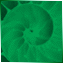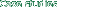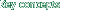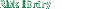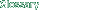PProduct Moment Correlation Coefficient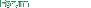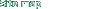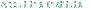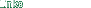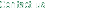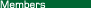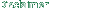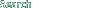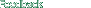### Product Moment Correlation Coefficient

 A statistic measuring the degree of linear relationship between two variables that have been measured on interval or ratio scales. It is calculated by multiplying the z scores of the two variables by one another and then calculating the mean of these products.